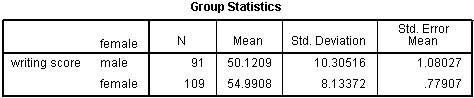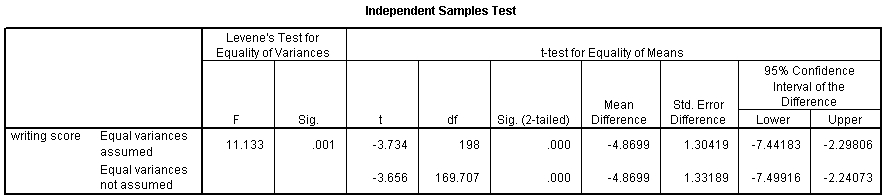## Two independent samples t-test

An independent samples t-test is used when you want to compare the means of a normally distributed interval dependent variable for two independent groups. For example, using the hsb2 data file, say we wish to test whether the mean for write is the same for males and females.

t-test groups = female(0 1) /variables = write.Because the standard deviations for the two groups are similar (10.3 and 8.1), we will use the “equal variances assumed” test. The results indicate that there is a statistically significant difference between the mean writing score for males and females (t = -3.734, p = .000). In other words, females have a statistically significantly higher mean score on writing (54.99) than males (50.12).